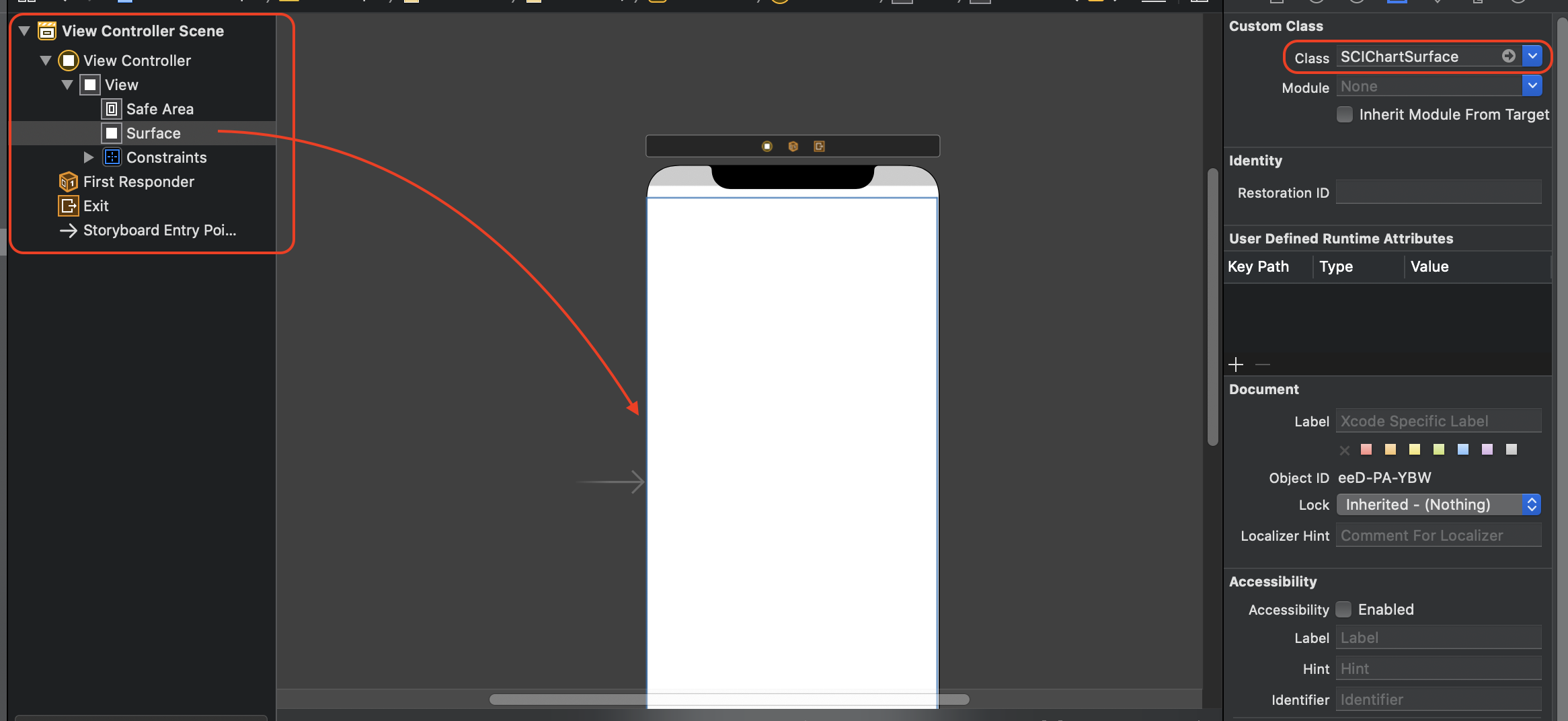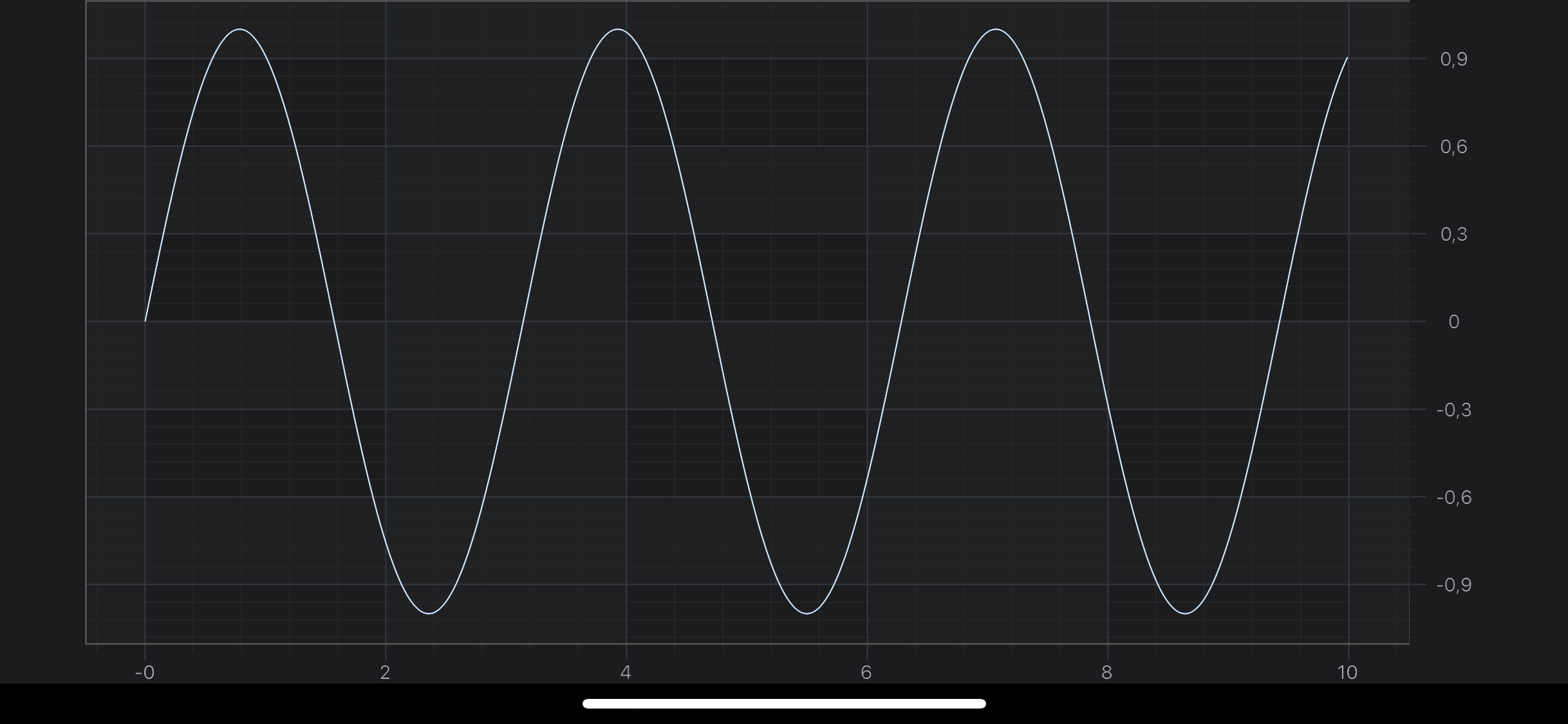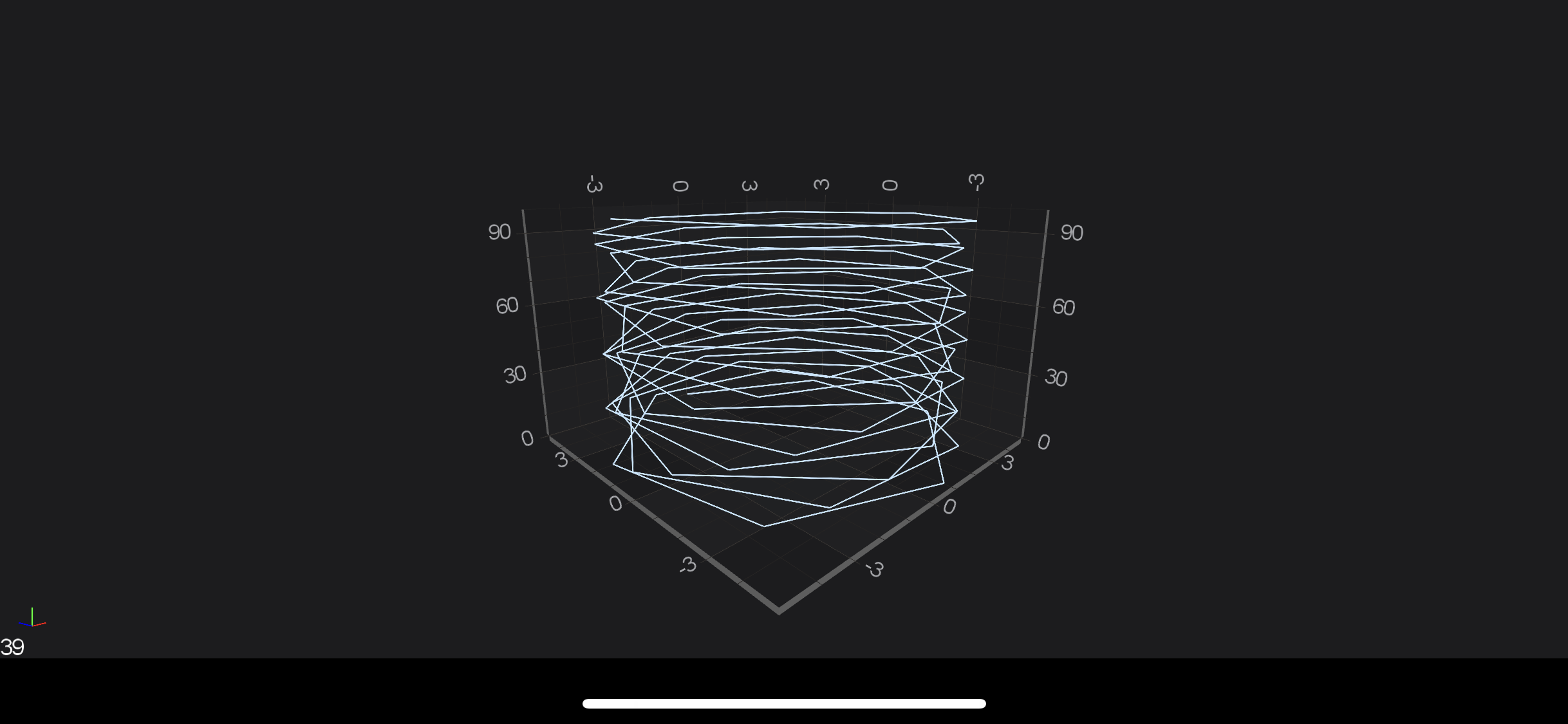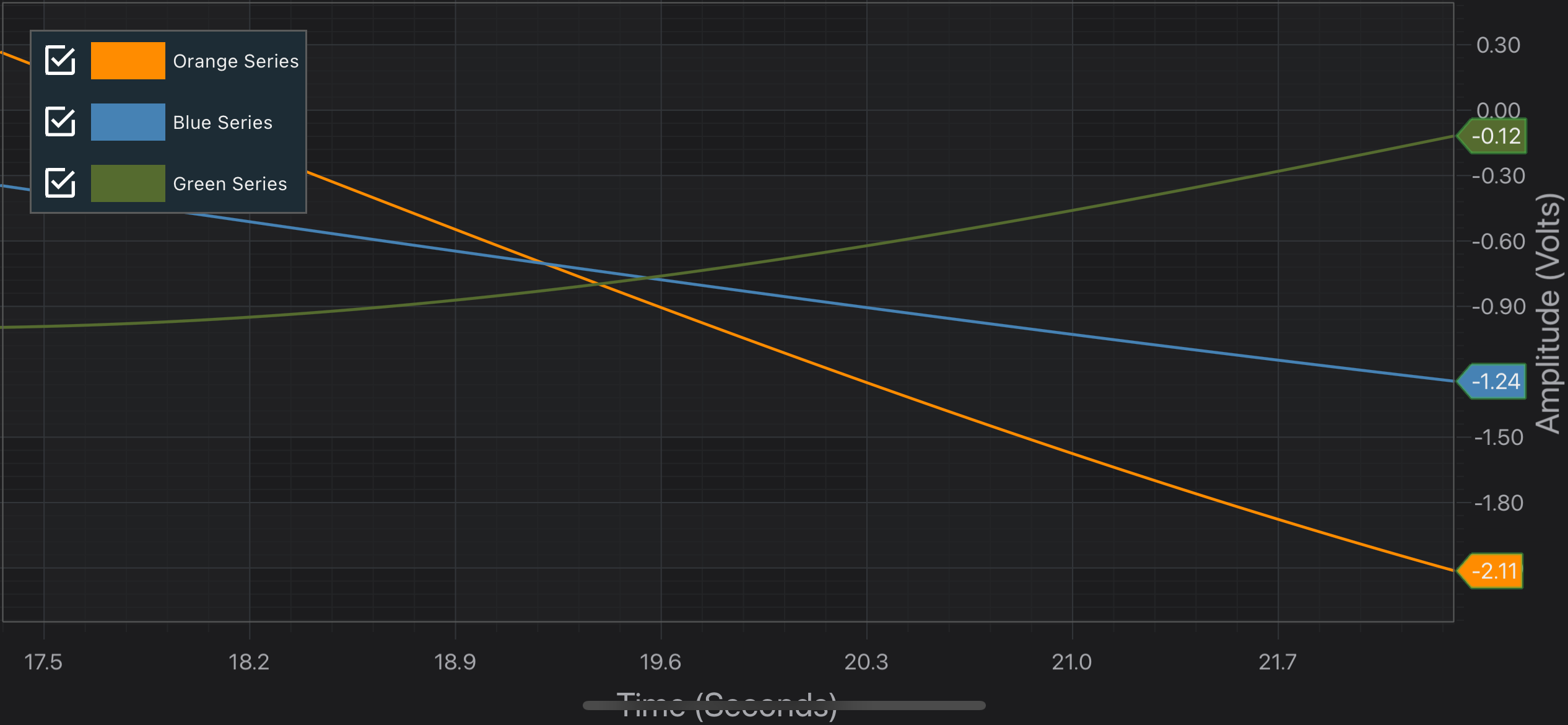iOS & macOS Charting Documentation - SciChart iOS & macOS Charts SDK v4.x

# Creating your first SciChart iOS App

Playing around with SciChart iOS Examples Suite is great, but you are probably interested in creating your own application by adding some charts! Let’s get started and create our first SciChart iOS App using Objective C.

## Setup XCode project

Assuming you’ve prepared development environment and created “Single View App” in either `Objective-C`, `Swift` or even `Xamarin.iOS` - next step should be integrating SciChart.framework. The easiest way of doing so is by using CocoaPods. Add the following to your Podfile, and then run `pod install`

``````source 'https://github.com/ABTSoftware/PodSpecs.git'
platform :ios, '8.0'

use_frameworks!
target 'YourTargetName' do
# Use the latest available Version
pod 'SciChart'
end
``````

NOTE: You can also get the extensive sample app downloading the SciChart iOS Trial package, which can be used for manual integration of SciChart.framework.

Before you build and run the application, you will need to apply a trial or purchased license key. You can find full instructions on the page Licensing SciChart iOS.

You can fetch a Trial License Key directly from the Downloads page following instructions from Licensing SciChart iOS. Or, if you have purchased SciChart iOS, you can find the full purchased license keys at your SciChart Account Page.

When you have your key, you should apply it via `+[SCIChartSurface setRuntimeLicenseKey:]` like below:

NOTE: The License Key must be set in your app once, and once only before any `SCIChartSurface` instance is initialized.

From here, you can create 2D or 3D chart. Please refer to the following sections for more information:

## The SCIChartSurface Type

The root 2D chart view is called the `SCIChartSurface`. This is the UIKit UIView you will be adding to your applications wherever you need a chart. You can add more than one `SCIChartSurface`, you can configure them independently, and you can link them together.

Since this is a Quick Start Example, we will use the one instance of `SCIChartSurface`, so let’s start by declaring one!

#### Declaring a SciChartSurface Instance

There few ways of adding `SCIChartSurface` to your application. We will look more closely into the following:

#### Adding SCIChartSurface via the .storyboard

Open up our `.storyboard` file. Add UIView onto the ViewController and set it’s class to the `SCIChartSurface`. Then add an IBOutlet for your SCIChartSurface in your ViewController code to be able to manipulate with it later on.#### Adding SCIChartSurface purely from code

In your ViewController you will need to import `SciChart` and instantiate the `SCIChartSurface`. See the code below:

#import <SciChart/SciChart.h> … - (void)viewDidLoad { [super viewDidLoad]; SCIChartSurface *surface = [SCIChartSurface new]; surface.translatesAutoresizingMaskIntoConstraints = NO; [self.view addSubview:surface]; [surface.topAnchor constraintEqualToAnchor:self.view.safeAreaLayoutGuide.topAnchor].active = YES; [surface.bottomAnchor constraintEqualToAnchor:self.view.safeAreaLayoutGuide.bottomAnchor].active = YES; [surface.leftAnchor constraintEqualToAnchor:self.view.leftAnchor].active = YES; [surface.rightAnchor constraintEqualToAnchor:self.view.rightAnchor].active = YES; }
import SciChart … override func viewDidLoad() { super.viewDidLoad() let surface = SCIChartSurface() surface.translatesAutoresizingMaskIntoConstraints = false self.view.addSubview(surface) surface.topAnchor.constraint(equalTo: self.view.safeAreaLayoutGuide.topAnchor).isActive = true surface.bottomAnchor.constraint(equalTo: self.view.safeAreaLayoutGuide.bottomAnchor).isActive = true surface.leftAnchor.constraint(equalTo: self.view.leftAnchor).isActive = true surface.rightAnchor.constraint(equalTo: self.view.rightAnchor).isActive = true }

#### Adding Axes to the SCIChartSurface

Once you have added a `SCIChartSurface` into your ViewController, you will not see anything drawn because you need to add axes. This is an important thing here - two axes X and Y has to be added to your surface. This is a bare minimum to see a drawn grid on your device.

Now, we would like to see something more than just an empty grid, e.g. Line Chart. So let’s add some RenderableSeries with appropriate DataSeries to our surface:

const int count = 1000; SCIDoubleValues *xValues = [[SCIDoubleValues alloc] initWithCapacity:count]; SCIDoubleValues *yValues = [[SCIDoubleValues alloc] initWithCapacity:count]; for (int i = 0; i < count; i++) { double x = 10.0 * i / count; double y = sin(2 * x); [xValues add:x]; [yValues add:y]; } id<ISCIXyDataSeries> dataSeries = [[SCIXyDataSeries alloc] initWithXType:SCIDataType_Double yType:SCIDataType_Double]; [dataSeries appendValuesX:xValues y:yValues]; id<ISCIRenderableSeries> rSeries = [SCIFastLineRenderableSeries new]; rSeries.dataSeries = dataSeries; [self.surface.renderableSeries add:rSeries];
let count = 1000 let xValues = SCIDoubleValues(capacity: count) let yValues = SCIDoubleValues(capacity: count) for i in 0 ..< count { let x: Double = 10.0 * Double(i) / Double(count) let y: Double = sin(2 * x) xValues.add(x) yValues.add(y) } let dataSeries = SCIXyDataSeries(xType: .double, yType: .double) dataSeries.append(x: xValues, y: yValues) let renderableSeries = SCIFastLineRenderableSeries() renderableSeries.dataSeries = dataSeries self.surface.renderableSeries.add(renderableSeries)
var count = 1000; var xValues = new SCIDoubleValues(count); var yValues = new SCIDoubleValues(count); for (int i = 0; i < count; i++) { var x = 10.0 * i / count; var y = Math.Sin(2 * x); xValues.Add(x); yValues.Add(y); } var dataSeries = new XyDataSeries(); dataSeries.AppendValues(xValues, yValues); var renderableSeries = new SCIFastLineRenderableSeries(); renderableSeries.DataSeries = dataSeries; Surface.RenderableSeries.Add(renderableSeries);

NOTE: You might have noticed, that we used `SCIDoubleValues` while appending points to `ISCIXyDataSeries`. That’s the recommended way of appending data, due to better performance, comparing to adding points one by one. You can use `-[ISCIXyDataSeries appendX:y:]` if you want though.

#### Final example code

So let’s see what we’ve managed to get. Let’s see the listing from the ViewController below:

#import “ViewController.h” #import <SciChart/SciChart.h> @interface ViewController () // Surface is added in Storyboard @property (weak, nonatomic) IBOutlet SCIChartSurface *surface; @end @implementation ViewController - (void)viewDidLoad { [super viewDidLoad]; id<ISCIAxis> xAxis = [SCINumericAxis new]; xAxis.growBy = [[SCIDoubleRange alloc] initWithMin:0.05 max:0.05]; id<ISCIAxis> yAxis = [SCINumericAxis new]; yAxis.growBy = [[SCIDoubleRange alloc] initWithMin:0.05 max:0.05]; const int count = 1000; SCIDoubleValues *xValues = [[SCIDoubleValues alloc] initWithCapacity:count]; SCIDoubleValues *yValues = [[SCIDoubleValues alloc] initWithCapacity:count]; for (int i = 0; i < count; i++) { double x = 10.0 * i / count; double y = sin(2 * x); [xValues add:x]; [yValues add:y]; } id<ISCIXyDataSeries> dataSeries = [[SCIXyDataSeries alloc] initWithXType:SCIDataType_Double yType:SCIDataType_Double]; [dataSeries appendValuesX:xValues y:yValues]; id<ISCIRenderableSeries> rSeries = [SCIFastLineRenderableSeries new]; rSeries.dataSeries = dataSeries; [SCIUpdateSuspender usingWithSuspendable:self.surface withBlock:^{ [self.surface.xAxes add:xAxis]; [self.surface.yAxes add:yAxis]; [self.surface.renderableSeries add:rSeries]; }]; } @end
import UIKit import SciChart class ViewController: UIViewController { // Surface is added in Storyboard @IBOutlet weak var surface: SCIChartSurface! override func viewDidLoad() { super.viewDidLoad() let xAxis = SCINumericAxis() xAxis.growBy = SCIDoubleRange(min: 0.05, max: 0.05) let yAxis = SCINumericAxis() yAxis.growBy = SCIDoubleRange(min: 0.05, max: 0.05) let count = 1000 let xValues = SCIDoubleValues(capacity: count) let yValues = SCIDoubleValues(capacity: count) for i in 0 ..< count { let x: Double = 10.0 * Double(i) / Double(count) let y: Double = sin(2 * x) xValues.add(x) yValues.add(y) } let dataSeries = SCIXyDataSeries(xType: .double, yType: .double) dataSeries.append(x: xValues, y: yValues) let renderableSeries = SCIFastLineRenderableSeries() renderableSeries.dataSeries = dataSeries SCIUpdateSuspender.usingWith(self.surface) { self.surface.xAxes.add(xAxis) self.surface.yAxes.add(yAxis) self.surface.renderableSeries.add(renderableSeries) } } }
public class ViewController : UIViewController { // Surface is added in Storyboard public SCIChartSurface Surface; public override void ViewDidLoad() { base.ViewDidLoad(); var xAxis = new SCINumericAxis { GrowBy = new SCIDoubleRange(0.05, 0.05) }; var yAxis = new SCINumericAxis { GrowBy = new SCIDoubleRange(0.05, 0.05) }; var count = 1000; var xValues = new SCIDoubleValues(count); var yValues = new SCIDoubleValues(count); for (int i = 0; i < count; i++) { var x = 10.0 * i / count; var y = Math.Sin(2 * x); xValues.Add(x); yValues.Add(y); } var dataSeries = new XyDataSeries(); dataSeries.AppendValues(xValues, yValues); var renderableSeries = new SCIFastLineRenderableSeries(); renderableSeries.DataSeries = dataSeries; using (Surface.SuspendUpdates()) { Surface.XAxes.Add(xAxis); Surface.YAxes.Add(yAxis); Surface.RenderableSeries.Add(renderableSeries); } } }

NOTE: Please note that we’ve added axes and renderableSeries to `SCIChartSurface` inside `+[SCIUpdateSuspender usingWithSuspendable:withBlock:]` block. This allows you to suspend surface instance, and refresh it only one time after you finished all needed operations. That’s highly recommended technique if you want to omit performance decrease due to triggering refreshes on every operation which could be performed in one batch.## The SCIChartSurface3D Type

The root 3D chart view is called the `SCIChartSurface3D`. This is the UIKit UIView which you will be adding to your applications wherever you need a 3D chart You can add more than one `SCIChartSurface3D`, you can configure them independently and you can link them together.

Since this is a Quick Start Example, we will use the one instance of `SCIChartSurface3D`, so let’s start by declaring one!

#### Declaring a SCIChartSurface3D Instance

Declaring `SCIChartSurface3D` to your application is no different than the regular SCIChartSurface, so please refer to the corresponding section in this article.

#### Adding 3D Axes to the SCIChartSurface3D

Once you have added a `SCIChartSurface3D` into your ViewController, you will not see anything drawn because you need to add axes. This is an important thing here - three axes X, Y, and Z has to be added to your surface. This is a bare minimum to see a drawn grid on your device.

self.surface.xAxis = [SCINumericAxis3D new]; self.surface.yAxis = [SCINumericAxis3D new]; self.surface.zAxis = [SCINumericAxis3D new];
self.surface.xAxis = SCINumericAxis3D() self.surface.yAxis = SCINumericAxis3D() self.surface.zAxis = SCINumericAxis3D()
Surface.XAxis = new SCINumericAxis3D(); Surface.YAxis = new SCINumericAxis3D(); Surface.ZAxis = new SCINumericAxis3D();

Now, we would like to see something more than just an empty grid, e.g. Scatter 3D Chart. So let’s add some RenderableSeries3D with appropriate DataSeries 3D to our surface:

SCIXyzDataSeries3D *dataSeries = [[SCIXyzDataSeries3D alloc] initWithXType:SCIDataType_Double yType:SCIDataType_Double zType:SCIDataType_Double]; for (int i = 0; i < 100; ++i) { double x = 5 * sin(i); double y = i; double z = 5 * cos(i); [dataSeries appendX:@(x) y:@(y) z:@(z)]; } SCIPointLineRenderableSeries3D *rSeries = [SCIPointLineRenderableSeries3D new]; rSeries.dataSeries = dataSeries; rSeries.strokeThickness = 3.0; [self.surface.renderableSeries add:rSeries];
let dataSeries = SCIXyzDataSeries3D(xType: .double, yType: .double, zType: .double) for i in 0 ..< 100 { let x = 5 * sin(Double(i)) let y = Double(i) let z = 5 * cos(Double(i)) dataSeries.append(x: x, y: y, z: z) } let rSeries = SCIPointLineRenderableSeries3D() rSeries.dataSeries = dataSeries rSeries.strokeThickness = 3.0 self.surface.renderableSeries.add(rSeries)
var dataSeries = new XyzDataSeries3D(); for (int i = 0; i < 100; i++) { double x = 5 * Math.Sin(i); double y = i; double z = 5 * Math.Cos(i); dataSeries.Append(x, y, z); } var rSeries = new SCIPointLineRenderableSeries3D { DataSeries = dataSeries, StrokeThickness = 3f }; Surface.RenderableSeries.Add(rSeries);

#### Final example 3D code

So let’s see what we’ve managed to get. Let’s see the listing from the ViewController below:

#import “ViewController.h” #import <SciChart/SciChart.h> @interface ViewController () // Surface is added in Storyboard @property (weak, nonatomic) IBOutlet SCIChartSurface3D *surface; @end @implementation ViewController - (void)viewDidLoad { [super viewDidLoad]; SCIXyzDataSeries3D *dataSeries = [[SCIXyzDataSeries3D alloc] initWithXType:SCIDataType_Double yType:SCIDataType_Double zType:SCIDataType_Double]; for (int i = 0; i < 100; ++i) { double x = 5 * sin(i); double y = i; double z = 5 * cos(i); [dataSeries appendX:@(x) y:@(y) z:@(z)]; } SCIPointLineRenderableSeries3D *rSeries = [SCIPointLineRenderableSeries3D new]; rSeries.dataSeries = dataSeries; rSeries.strokeThickness = 3.0; [SCIUpdateSuspender usingWithSuspendable:self.surface withBlock:^{ self.surface.xAxis = [SCINumericAxis3D new]; self.surface.yAxis = [SCINumericAxis3D new]; self.surface.zAxis = [SCINumericAxis3D new]; [self.surface.renderableSeries add:rSeries]; }]; } @end
import UIKit import SciChart class ViewController: UIViewController { // Surface is added in Storyboard @IBOutlet weak var surface: SCIChartSurface3D! override func viewDidLoad() { super.viewDidLoad() let dataSeries = SCIXyzDataSeries3D(xType: .double, yType: .double, zType: .double) for i in 0 ..< 100 { let x = 5 * sin(Double(i)) let y = Double(i) let z = 5 * cos(Double(i)) dataSeries.append(x: x, y: y, z: z) } let rSeries = SCIPointLineRenderableSeries3D() rSeries.dataSeries = dataSeries rSeries.strokeThickness = 3.0 SCIUpdateSuspender.usingWith(self.surface) { self.surface.xAxis = SCINumericAxis3D() self.surface.yAxis = SCINumericAxis3D() self.surface.zAxis = SCINumericAxis3D() self.surface.renderableSeries.add(rSeries) } } }
public class ViewController : UIViewController { // Surface is added in Storyboard public SCIChartSurface3D Surface; public override void ViewDidLoad() { base.ViewDidLoad(); var dataSeries = new XyzDataSeries3D(); for (int i = 0; i < 100; i++) { double x = 5 * Math.Sin(i); double y = i; double z = 5 * Math.Cos(i); dataSeries.Append(x, y, z); } var rSeries = new SCIPointLineRenderableSeries3D { DataSeries = dataSeries, StrokeThickness = 3f }; using (Surface.SuspendUpdates()) { Surface.XAxis = new SCINumericAxis3D(); Surface.YAxis = new SCINumericAxis3D(); Surface.ZAxis = new SCINumericAxis3D(); Surface.RenderableSeries.Add(rSeries); } } }

NOTE: Please note that we’ve added axes and renderableSeries to `SCIChartSurface3D` inside `+[SCIUpdateSuspender usingWithSuspendable:withBlock:]` block. This allows you to suspend surface instance, and refresh it only one time after you finished all needed operations. That’s highly recommended technique if you want to omit performance decrease due to triggering refreshes on every operation which could be performed in one batch.## Adding SCIChartSurface to SwiftUI App

Since `SCIChartSurface` is an UIKit UIView under the hood, you can use UIViewRepresentable protocol to integrate our `SCIChartSurface` into a SwiftUI view hierarchy. There are two approaches here:

#### Configure SCIChartSurface all in one place

We begin by declaring a SurfaceView struct, which conforms to the UIViewRepresentable protocol. Import SciChart, instantiate a `SCIChartSurface`, add axes, renderable series, and other elements to your `SCIChartSurface` as described above. Your final code will look something like this:

import SwiftUI import SciChart struct SurfaceView: UIViewRepresentable { func makeUIView(context: Context) -> SCIChartSurface { let surface = SCIChartSurface() let xAxis = SCINumericAxis() xAxis.growBy = SCIDoubleRange(min: 0.05, max: 0.05) let yAxis = SCINumericAxis() yAxis.growBy = SCIDoubleRange(min: 0.05, max: 0.05) let count = 1000 let xValues = SCIDoubleValues(capacity: count) let yValues = SCIDoubleValues(capacity: count) for i in 0 ..< count { let x: Double = 10.0 * Double(i) / Double(count) let y: Double = sin(2 * x) xValues.add(x) yValues.add(y) } let dataSeries = SCIXyDataSeries(xType: .double, yType: .double) dataSeries.append(x: xValues, y: yValues) let renderableSeries = SCIFastLineRenderableSeries() renderableSeries.dataSeries = dataSeries SCIUpdateSuspender.usingWith(self.surface) { self.surface.xAxes.add(xAxis) self.surface.yAxes.add(yAxis) self.surface.renderableSeries.add(renderableSeries) } return surface } func updateUIView(_ uiView: SCIChartSurface, context: Context) {} }

Next, place your SurfaceView instance into your ContentView body, same as any other SwiftUI view, like this:

struct ContentView: View { var body: some View { SurfaceView() } }

#### SwiftUI helper functions

In the example above, we configure `SCIChartSurface` the old-fashioned imperative way. But you can turn this code into a set of more readable declarative functions, so it looks similar to any other SwiftUI view modifier.

Let’s start by wrapping `SCIChartSurface` into a UIViewRepresentable protocol. Here you can find a generic wrapper for any UIKit view that we will use for our `SCIChartSurface`:

import SwiftUI struct UIViewRepresentableWrapper: UIViewRepresentable { typealias Updater = (Wrapped, Context) -> Void var makeView: () -> Wrapped var updateView: (Wrapped, Context) -> Void init(makeView: @escaping () -> Wrapped, updateView: @escaping Updater) { self.makeView = makeView self.updateView = updateView } func makeUIView(context: Context) -> Wrapped { makeView() } func updateUIView(_ view: Wrapped, context: Context) { updateView(view, context) } } extension UIViewRepresentableWrapper { init( makeView: @escaping @autoclosure () -> Wrapped, updateView: @escaping (Wrapped) -> Void ) { self.makeView = makeView self.updateView = { view, _ in updateView(view) } } init(makeView: @escaping @autoclosure () -> Wrapped) { self.makeView = makeView self.updateView = { _, _ in } } }

Next, we create a SCIChartSurfaceView with a few convenience initializers that you can use depending on your needs:

import SwiftUI import SciChart struct SCIChartSurfaceView: View { private var xAxes = SCIAxisCollection() private var yAxes = SCIAxisCollection() private var renderableSeries = SCIRenderableSeriesCollection() private var chartModifiers = SCIChartModifierCollection() private var makeSurface: (() -> SCIChartSurface)! private var updateSurface: ((SCIChartSurface) -> Void)! var body: some View { UIViewRepresentableWrapper( makeView: makeSurface(), updateView: { updateSurface(\$0) } ) } } extension SCIChartSurfaceView { init( makeSurface: @escaping () -> SCIChartSurface, updateSurface: @escaping (SCIChartSurface) -> Void ) { self.makeSurface = makeSurface self.updateSurface = { surface in updateSurface(surface)} } init(updateSurface: @escaping (SCIChartSurface) -> Void) { self.makeSurface = makeDefaultSurface self.updateSurface = { surface in updateSurface(surface) } } init(makeSurface: @escaping @autoclosure () -> SCIChartSurface) { self.init( makeSurface: makeSurface, updateSurface: { _ in } ) } } extension SCIChartSurfaceView { func makeDefaultSurface() -> SCIChartSurface { let surface = SCIChartSurface(frame: CGRect(x: 0, y: 0, width: 1, height: 1)) SCIUpdateSuspender.usingWith(surface) { surface.xAxes = xAxes surface.yAxes = yAxes surface.renderableSeries = renderableSeries surface.chartModifiers = chartModifiers } return surface } }

The last piece of the puzzle is creating a few SCIChartSurfaceView extensions that will modify `SCIChartSurface` properties - X-Axes, Y-Axes, Renderable Series, and Chart Modifiers:

extension SCIChartSurfaceView { func xAxis(_ axis: ISCIAxis) -> SCIChartSurfaceView { xAxes.add(axis) return self } func yAxis(_ axis: ISCIAxis) -> SCIChartSurfaceView { yAxes.add(axis) return self } func renderableSeries(_ rs: ISCIRenderableSeries) -> SCIChartSurfaceView { renderableSeries.add(rs) return self } func modifier(_ modifier: ISCIChartModifier) -> SCIChartSurfaceView { chartModifiers.add(modifier) return self } }

With all those helper functions, `SCIChartSurface` configuring will look like the following:

import SwiftUI import SciChart struct ContentView: View { @ObservedObject var viewModel = ChartViewModel() var body: some View { SCIChartSurfaceView(updateSurface: { surface in // update surface properties on viewModel change if needed }) .xAxis(xAxis) .yAxis(yAxis) .renderableSeries(rSeries1) .renderableSeries(rSeries2) .renderableSeries(rSeries3) .modifier(legendModifier) .modifier(SCISeriesValueModifier()) .ignoresSafeArea() } } extension ContentView { private var xAxis: ISCIAxis { let xAxis = SCINumericAxis() xAxis.autoRange = .always xAxis.axisTitle = “Time (Seconds)” xAxis.textFormatting = “0.0” return xAxis } private var yAxis: ISCIAxis { let yAxis = SCINumericAxis() yAxis.autoRange = .always yAxis.axisTitle = “Amplitude (Volts)” yAxis.growBy = SCIDoubleRange(min: 0.1, max: 0.1) yAxis.textFormatting = “0.00” yAxis.cursorTextFormatting = “0.00” return yAxis } } extension ContentView { private var rSeries1: ISCIRenderableSeries { let rSeries = SCIFastLineRenderableSeries() rSeries.dataSeries = viewModel.chartModel.ds1 rSeries.strokeStyle = SCISolidPenStyle(color: 0xFFFF8C00, thickness: 2) return rSeries } private var rSeries2: ISCIRenderableSeries { let rSeries = SCIFastLineRenderableSeries() rSeries.dataSeries = viewModel.chartModel.ds2 rSeries.strokeStyle = SCISolidPenStyle(color: 0xFF4682B4, thickness: 2) return rSeries } private var rSeries3: ISCIRenderableSeries { let rSeries = SCIFastLineRenderableSeries() rSeries.dataSeries = viewModel.chartModel.ds3 rSeries.strokeStyle = SCISolidPenStyle(color: 0xFF556B2F, thickness: 2) return rSeries } } extension ContentView { private var legendModifier: ISCIChartModifier { let legendModifier = SCILegendModifier() legendModifier.margins = UIEdgeInsets(top: 16, left: 16, bottom: 16, right: 16) return legendModifier } }NOTE: The full SwiftUIShowcase example can be found on the GitHub